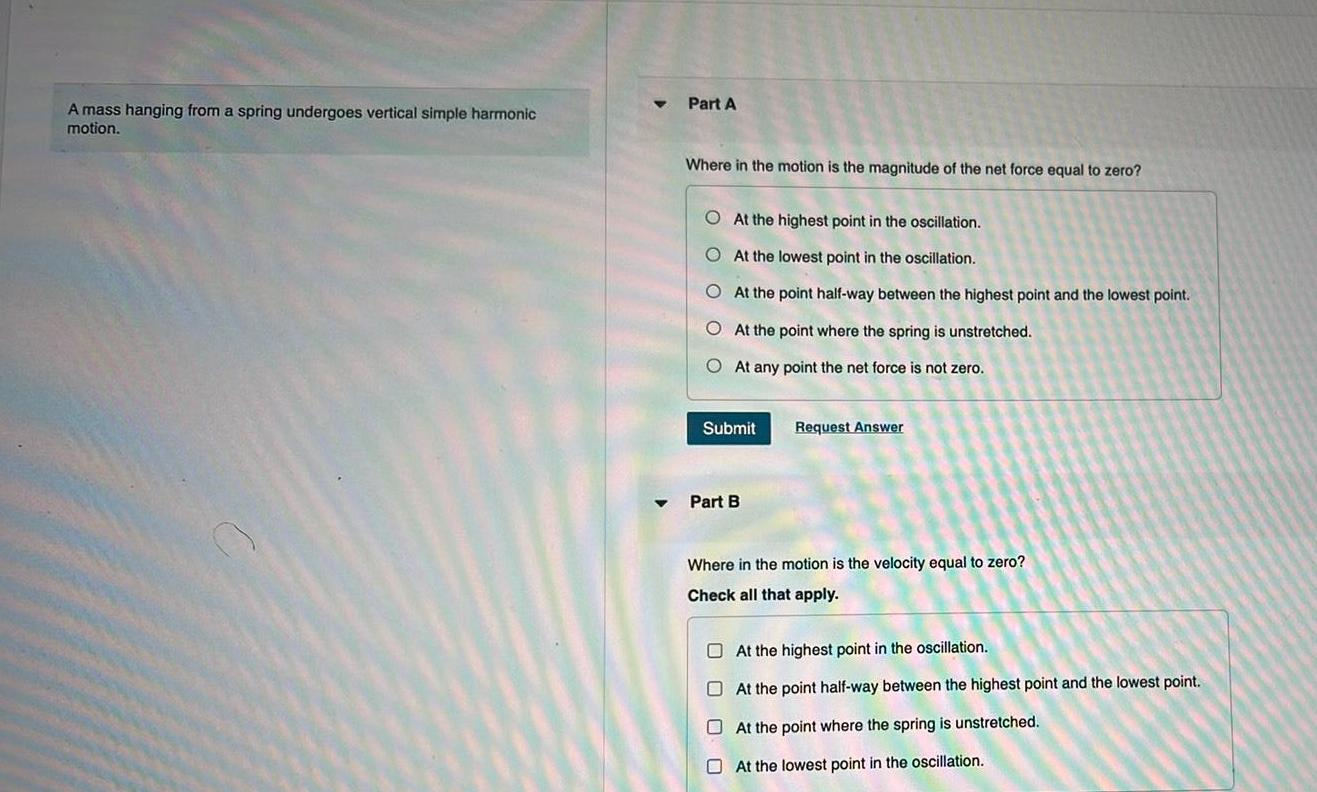Question:

# A mass hanging from a spring undergoes vertical simple

Last updated: 8/3/2022A mass hanging from a spring undergoes vertical simple harmonic motion. Where in the motion is the magnitude of the net force equal to zero? At the highest point in the oscillation. At the lowest point in the oscillation. At the point half-way between the highest point and the lowest point. At the point where the spring is unstretched. At any point the net force is not zero. Where in the motion is the velocity equal to zero? Check all that apply. At the highest point in the oscillation. At the point half-way between the highest point and the lowest point. At the point where the spring is unstretched. At the lowest point in the oscillation.